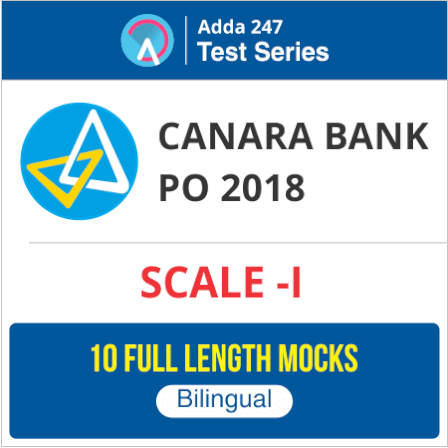Dear Aspirants,

### Reasoning Questions for IBPS Clerk 2018

Reasoning Ability is an onerous section. With the increasing complexity of questions, it becomes hard for one to give it the cold shoulder. The only way to make the grade in this particular section in the forthcoming banking exams like IBPS Clerk Prelims is to practice continuously with all your heart and soul. And, to let you practice with the best of the latest pattern questions, here is the Adda247 Reasoning Quiz based on the exact same pattern of questions that are being asked in the exams.

### Watch Video Solution

Directions (1-4): Study the information carefully and answer the questions given below.

Eight persons A, B, C, D, E, F, G and H have different height. G is not 3rd shortest. D is not 5th tallest. Only two persons are taller than C. E is taller than F but shorter than D. H is the shortest. D is taller than G but shorter than A and C. A is not the 2nd tallest. E is not 3rd shortest.

Q1. Who among the following is 2nd tallest?

E
F
C
B
none of these
Solution:
A > B > C > D > E > F > G > H

Q2. How many persons are shorter than F?
Two
One
Three
Four
None of these
Solution:
A > B > C > D > E > F > G > H

Q3. Who among the following is just taller to E?
D
F
C
B
none of these
Solution:
A > B > C > D > E > F > G > H

Q4. Who among the following is 5th shortest?
E
F
C
B
none of these
Solution:
A > B > C > D > E > F > G > H

Directions (5-8): Study the information carefully and answer the questions given below.

Eight persons are sitting in two parallel rows such that each row consists of four persons. Persons sitting in row 1 face persons sitting in row 2. D sits Diagonally opposite to H. G faces F. the number of persons sitting between A and C are same as between F and B. E is not sitting at any end. C and G do not face north. The one who faces E sits 2nd left to A. A and H are not in same row.

Q5. How many persons sit between A and D?

Two
One
cant be determined
same as between F and H
None of these
Q6. Who among the following faces E?
A
F
C
B
none of these

Q7. Which of the following statement is true regarding B ?
E faces opposite direction as B
F does not sits in same row as B
C faces B
B sits at one of the ends
none of these

Q8. Who among the following faces H?
E
F
C
B
none of these

Directions (9-10): Study the following information and answer the given questions.
In a family of seven members P is the father of Q, who is grandchild of R. T is married to P. G is paternal grandmother of D, who is brother of Q. S is sister-in-law of T. R is father in law of T.

Q9. How is S related to D?

Mother
Aunt
Sister
Cousin
Cannot be determined
Q10. How is Q related to G?
Son
Daughter
Grandson
Granddaughter
Cannot be determined

Directions (11-15): Study the following number sequence and answer the questions following it.

4 5 2 7 8 2 9 7 2 1 5 6 8 1 3 2 5 4 7 6 8 9 6 1 3 5 2 8 4 4

Q11. How many even numbers are there in the numeric series which are immediately preceded by a number, which is a perfect square?

One
Two
Three
More than three
None of these
Solution:
96,44

Q12. If all the odd numbers are dropped from the series, which number will be fifth to the right of seventh number from the left end?
2
8
6
4
None of these
Solution:
6

Q13. If 1 is replaced by 2 and 4 is replaced by 5 in the given number series, then which number will be fourth to the left of seventeenth number from the right end?
1
2
3
5
6
Solution:
2

Q14. If the position of the 1st and the 16th number, the 2nd and the 17th number, and so on up to the 15th and the 30th number are interchanged, than which number will be 8th to the right of 17th number from the right end?
5
6
8
9
None of these
Solution:
9

Q15. How many total odd number/s which is/are immediately preceded by a ‘perfect square’ are there in the above sequence?
Four
Five
Three
Seven
None of these
Solution:
Six- 45,97,15,13,13,47

You may also like to Read: